• iir数字滤波器matlab代码
千次阅读
2021-04-20 08:22:35

低通滤波器的设计：

1、基本指标

低通滤波器顾名思义就是低频率成分通过，高频率成分截止，那么在设计一个低通滤波器时首先要明白想要截止多大的频率。比如想截掉4Hz以上的信号，理想状态下就是将4Hz以上的信号成分全部截止，4Hz以下的信号全部保留。然而事实上几乎不存在这样的滤波器，通常情况下总是在通过频率和截止频率之间存在一个过渡带。通过频率这部分称为通带，允许通过的最大频率为通带截止频率ωp，截至频率这部分称为阻带，阻带最小截至频率为ωs，通带和阻带之间的部分为过渡带，也即ωp~ωp。通带之间的波动称为通带波动δp，阻带之间的波动称为阻带波动δs如图所示：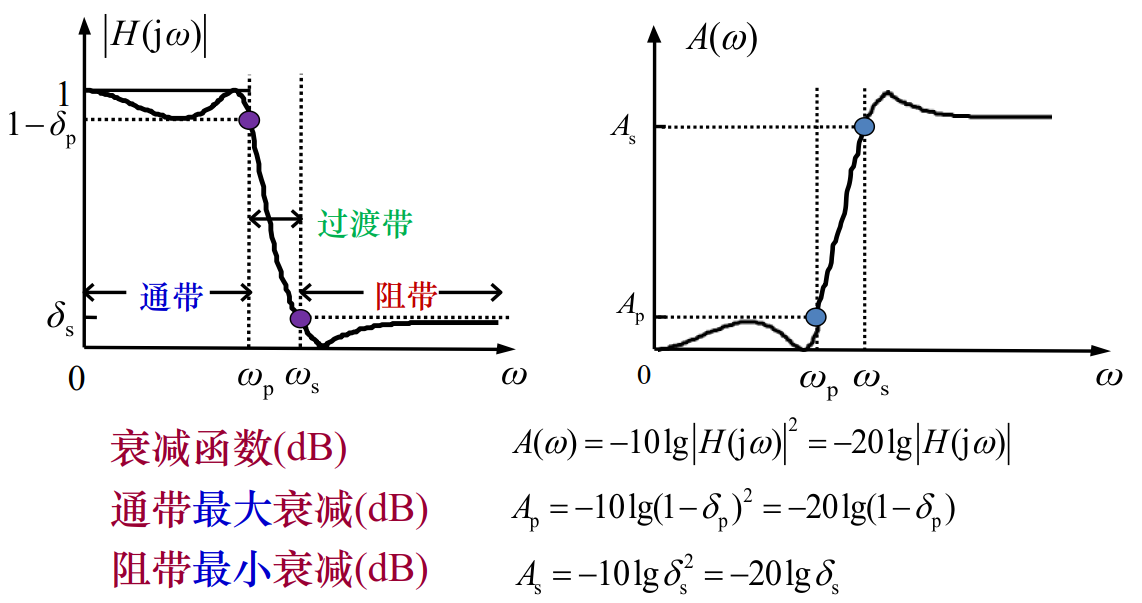2、Matlab函数介绍

声明：上面一节的基本指标均为模拟滤波器状态下的指标，Matlab不仅提供了模拟滤波器设计函数，同样提供了数字滤波器的设计函数，只是参数之间需要一些转换。

数字滤波器设计中，Wp和Ws为归一化角频率，如果工程要求设计低通数字滤波器满足通带截止频率为fp = 40，阻带截止频率fs = 50，则fp，fs和Wp，Ws之间的转换关系为：

Wp = 2*pi*fp/Fs;

Ws = 2*pi*fs/Fs;

截止频率的定义是当输出幅值响应下降到输入幅值的-3dB ()，也就是0.707(也就是)时对应的频率，也称3db截频

巴特沃斯数字滤波器函数：

[n,Wc] = buttord(Wp/pi,Ws/pi,Ap,As);

[b,a] = butter(n,Wc,'low');%低通滤波器

Ap和As的计算用上图中的公式计算获得。

function FilteredSignal = filtered(signal,Fs,fp,fs,Ap,As,FilterType)

% fp=40; fs=50; Ap=1; As=20;

%=============================================

% Fs:信号的频率

% fp：滤波器的通带频率

% fs:滤波器的截止频率

% Ap：通带最大衰减

% As：阻带最小衰减

% FilterType:滤波器类型，'low',低通，'high',高通

% FilteredSignal：滤波后的信号

%==============================================

Wp = 2*pi*fp/Fs;

Ws = 2*pi*fs/Fs;

[n,Wc] = buttord(Wp/pi,Ws/pi,Ap,As);

[b,a] = butter(n,Wc,FilterType);

omega=[Wp Ws];

h = freqz(b,a,omega); %Compute Ap and As of AF

fprintf('Ap= %.4f\n',-20*log10(abs(h(1))));

fprintf('As= %.4f\n',-20*log10(abs(h(2))));

FilteredSignal = filter(b,a,signal);

当 fp=40; fs=50; Ap=1; As=20;时，通过上面的代码设计的滤波器Ap= 0.9998，As= 20.0000，满足要求，设计的滤波器如下图：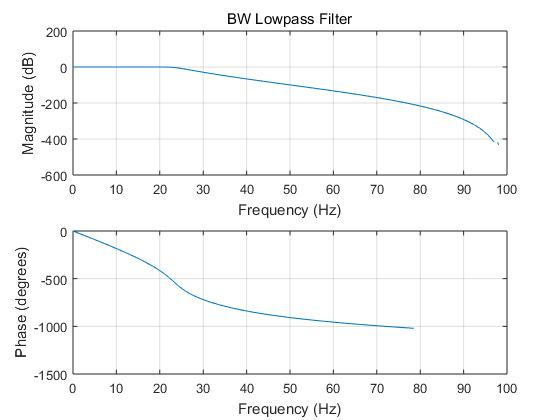切比雪夫I型数字滤波器函数：

[N,wc] = cheb1ord(Wp/pi,Ws/pi,Ap,As);

[b,a] = cheby1(N,Ap,wc,'low');

%% 切比雪夫1型低通滤波器

fp=40; fs=50; Ap=1; As=20;

Wp = 2*pi*fp/Fs;

Ws = 2*pi*fs/Fs;

[N,wc] = cheb1ord(Wp/pi,Ws/pi,Ap,As);

[b,a] = cheby1(N,Ap,wc,'low');

omega = [Wp,Ws];

h = freqz(b,a,omega);

fprintf('N = %.2f\n',N);

fprintf('Ap=%.4f\n',-20*log10(abs(h(1))));

fprintf('As=%.4f\n',-20*log10(abs(h(2))));

figure(7)

freqz(b,a,512,200);title('CBI Lowpass Filter')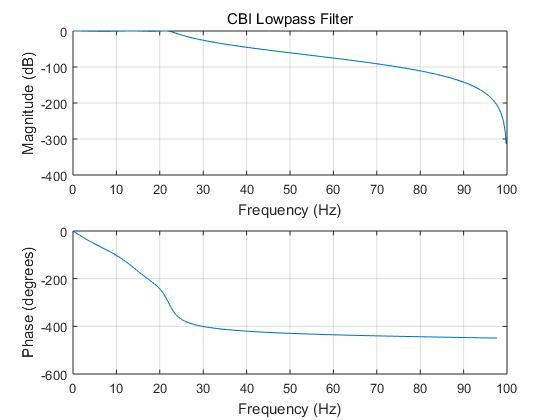N = 5.00；Ap=1.0000<=1；As=20.0093>20满足定义的Ap和As，通带刚好，阻带有余量。

%% 椭圆低通滤波器

[N,wc] = ellipord(Wp/pi,Ws/pi,Ap,As);

[b,a] = ellip(N,Ap,As,wc,'low');

omega = [Wp,Ws];

h = freqz(b,a,omega);

fprintf('N = %.2f\n',N);

fprintf('Ap=%.4f\n',-20*log10(abs(h(1))));

fprintf('As=%.4f\n',-20*log10(abs(h(2))));

figure

freqz(b,a,512,200);title('elip Lowpass Filter')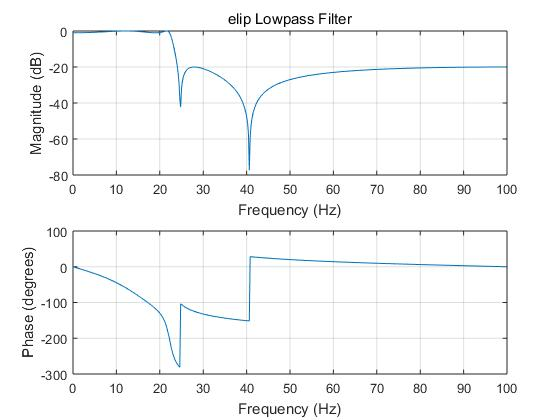更多相关内容
• iir数字滤波器matlab代码红外过滤器 MATLAB 代码，用于使用双线性变换技术设计 IIR Butterworth 低通数字滤波器，以满足以下规范。 假设 T=1 秒。 0.8<|H(e^jw)|<1，对于 0<w<0.2 pi H(e^jw)<0.2，...
• matlab代码，直接运行出结果，含以下几个部分的代码： 1. 巴特沃兹带通滤波器设计 2.带阻滤波器设计 3.递归型滤波器设计 4.对信号进行数字滤波
• 本压缩包内包含了IIR数字滤波器设计的实现代码，可以用各类窗函数实现IIR数字滤波器的设计
• 大连理工大学实验报告 学院(系)电子信息与电气工程学部 专业:电子信息工程 班级: 姓 名 学号 组 实验时间 实验室 大黑楼C221 实验台 指导教师签字 成绩 实验名称 实验三IIR数字滤波器设计 一实验目的和要求 掌握双线...
• 对含有1200hz和4800hz频率成分的信号进行了滤波，滤除了4800hz的信号
• IIR巴特沃斯滤波器实现（内含完整的MATLAB代码、CCS3.3软件仿真代码以及DSP实习报告）
• 1.设计IIR巴特沃斯低通滤波器2.将滤波器用于加噪声信号的处理3.改变参数指标比较不同
• 1．加深对IIR数字滤波器常用指标的理解； 2．学会设计IIR数字滤波器； 3．根据指标要求设计数字滤波器，并进行信号的处理。 二、实验原理： 1.脉冲响应不变法 MATLAB提供impinvar(num,den,Fs)函数，可以实现利用脉冲...

# 一、实验目的：

1．加深对IIR数字滤波器常用指标的理解；
2．学会设计IIR数字滤波器；
3．根据指标要求设计数字滤波器，并进行信号的处理。

# 二、实验原理：

## 1.脉冲响应不变法

MATLAB提供impinvar(num,den,Fs)函数，可以实现利用脉冲响应不变法将模拟滤波器转换为数字滤波器，其调用形式为：
[numd,dend]=impinvar(num,den,Fs)
式中num和den分别表示模拟滤波器系统函数H（s）的分子多项式系统和分母多项式系数，Fs是脉冲响应不变法中的抽样频率，单位是Hz。输出变量numd和dend分别表示数字滤波器系统函数H（z）的分子多项式系统和分母多项式系数。
【例6.1】利用BW型低通滤波器及脉冲响应不变法设计满足下列指标的数字滤波器。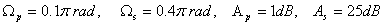程序如下：

     clear all
Wp=0.1*pi;
Ws=0.4*pi;
Ap=1;
As=25;
T=1;
Fs=1/T;
wp=Wp*Fs;
ws=Ws*Fs;
N=buttord(wp,ws,Ap,As,'s');
wc=wp/(10^(0.1*Ap)-1)^(1/2/N);
[num den]=butter(N,wc,'s');
[numd,dend]=impinvar(num,den,Fs);
w=linspace(0,pi,512);
h=freqz(numd,dend,w);
plot(w/pi,abs(h));
title('脉冲响应不变法');
%计算所设计滤波器的Ap和As
w1=[Wp,Ws];
h1=freqz(numd,dend,w1);
fprintf('Ap= %.4f\n',-20*log10(abs(h1(1))));
fprintf('As= %.4f\n',-20*log10(abs(h1(2))));


运行结果：
Ap= 0.9991
As= 30.3245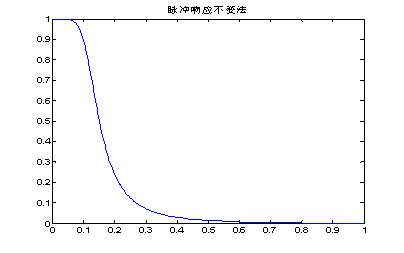图1 数字低通滤波器的幅度响应


## 2.双线性变换法

MATLAB提供bilinear(num,den,Fs)函数，可以实现利用双线性变换法将模拟滤波器转换为数字滤波器，其调用形式为：
[numd,dend]=bilinear(num,den,Fs)
式中num和den分别表示模拟滤波器系统函数H（s）的分子多项式系统和分母多项式系数，Fs=1/T。输出变量numd和dend分别表示数字滤波器系统函数H（z）的分子多项式系统和分母多项式系数。
【例6.2】利用CB1型滤波器及双线性变换法设计满足下列指标的数字高通滤波器。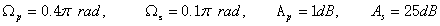程序如下：

		clear all
Wp=0.4*pi;
Ws=0.1*pi;
Ap=1;
As=25;
T=2;
Fs=1/T;
wp=(2/T)*tan(Wp/2);
ws=(2/T)*tan(Ws/2);
[N,wc]=cheb1ord(wp,ws,Ap,As,'s');
[num den]=cheby1(N,Ap,wc,'high','s');
[numd,dend]=bilinear(num,den,Fs);
w=linspace(0,pi,512);
h=freqz(numd,dend,w);
plot(w/pi,abs(h));
title('双线性变换法')
%计算所设计滤波器的Ap和As
w1=[Wp,Ws];
h1=freqz(numd,dend,w1);
fprintf('Ap= %.4f\n',-20*log10(abs(h1(1))));
fprintf('As= %.4f\n',-20*log10(abs(h1(2))));


运行结果：
Ap= 1.0000
As= 26.4153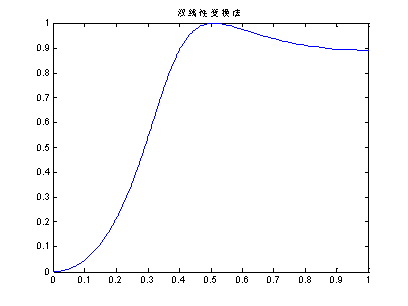图2 数字高通滤波器的幅度响应


## 3.数字滤波函数

（1）filter函数用来实现数字滤波器对数据的滤波，函数调用格式为：
y=filter(numd,dend,x)
其中，numd和dend分别为滤波器系统函数H（z）的分子和分母多项式的系数，x为滤波器的输入，y为滤波器的输出，y与x具有相同大小的向量。
（2）filtfilt函数实现零相位前后与后向结合滤波，其调用格式为：
y=filtfilt(numd,dend,x)
其中，numd和dend分别为滤波器系统函数H（z）的分子和分母多项式的系数，x为滤波器的输入，y为滤波器的输出，y与x具有相同大小的向量，这个函数实现的滤波后其输出信号与输入信号的相位一致，也就是没有改变信号波形形状。但filter函数滤波后有一些延迟，改变了信号的形状。

# 三、作业：

(1)示例代码：

clear all
Wp=0.2*pi;
Ws=0.3*pi;
Ap=1;
As=15;
T=0.01;
Fs=1/T;
wp=Wp*Fs;
ws=Ws*Fs;
N=buttord(wp,ws,Ap,As,'s');
wc=wp/(10^(0.1*Ap)-1)^(1/2/N);
[num den]=butter(N,wc,'s');
[numd,dend]=impinvar(num,den,Fs);
w=linspace(0,pi,512);
h=freqz(numd,dend,w);
plot(w/pi,abs(h));
title('脉冲响应不变法');
%计算所设计滤波器的Ap和As
w1=[Wp,Ws];
h1=freqz(numd,dend,w1);
fprintf('Ap= %.4f\n',-20*log10(abs(h1(1))));
fprintf('As= %.4f\n',-20*log10(abs(h1(2))));


（2）运行结果：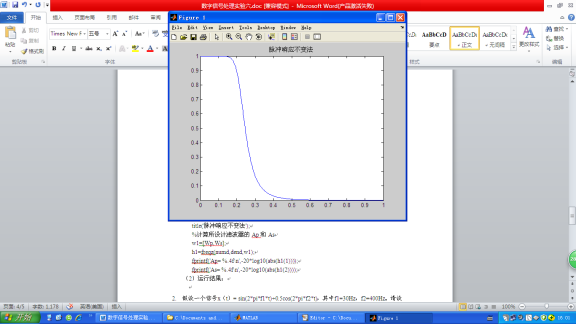2.假设一个信号x（t）= sin(2pif1t)+0.5cos(2pif2t)，其中f1=30Hz，f2=400Hz。请设计一个IIR数字滤波器，将f2滤除掉。请写出程序，并画出原信号与原信号通过滤波器的输出信号的图形。（数字低通滤波器）
（1）示例代码：

clear all
Wp=0.075*pi;
Ws=1*pi;
Ap=1;
As=25;
T=0.01;
Fs=1/T;
wp=Wp*Fs;
ws=Ws*Fs;
N=buttord(wp,ws,Ap,As,'s');
wc=wp/(10^(0.1*Ap)-1)^(1/2/N);
[num den]=butter(N,wc,'s');
[numd,dend]=impinvar(num,den,Fs);

w=linspace(0,pi,512);
h=freqz(numd,dend,w);
plot(w/pi,abs(h));
title('脉冲响应不变法');
%计算所设计滤波器的Ap和As
w1=[Wp,Ws];
h1=freqz(numd,dend,w1);
fprintf('Ap= %.4f\n',-20*log10(abs(h1(1))));
fprintf('As= %.4f\n',-20*log10(abs(h1(2))));
%信号传输
fs=800;
dt=1/fs;   %模拟信号采样间隔
f1=30;f2=400;
t=0:dt:1;
x=sin(2*pi*f1*t)+0.5*cos(2*pi*f2*t);
y=filter(numd,dend,x); % 模拟输出
L=length(x);
x1=fftshift(fft(x));
ff=(-L/2:L/2-1)*fs/L;
y1=fftshift(fft(y));
figure(2)
subplot(2,2,1)
plot(t,x);
xlabel ('(a)输入信号');
subplot(2,2,2)
plot(ff,abs(x1));
xlabel ('(b)输入信号频谱');
subplot(2,2,3)
plot(t,y);
xlabel('(c)输出信号');
subplot(2,2,4)
plot(ff,abs(y1));
xlabel ('(d)输出信号频谱'


（2）运行结果：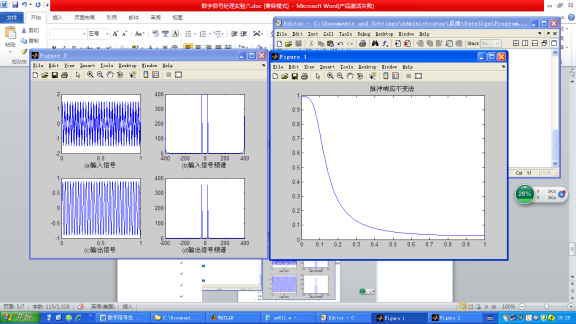3.假设一个信号x（t）= sin(2pif1t)+0.5cos(2pif2t)，其中f1=10Hz，f2=100Hz。请设计一个IIR数字滤波器能把f1滤除掉，请写出程序，并画出原信号与原信号通过滤波器的输出信号的图形。
（1）示例代码：

clear all
Wp=0.1*pi;
Ws=0.2*pi;
Ap=1;
As=25;
T=1;
Fs=1/T;
wp=(2/T)*tan(Wp/2);
ws=(2/T)*tan(Ws/2);
[N,wc]=cheb1ord(wp,ws,Ap,As,'s');
[num den]=butter(N,wc,'s');
[numd,dend]=impinvar(num,den,Fs);
w=linspace(0,pi,512);
h=freqz(numd,dend,w);
plot(w/pi,abs(h));
title('脉冲响应不变法');
%计算所设计滤波器的Ap和As
w1=[Wp,Ws];
h1=freqz(numd,dend,w1);
fprintf('Ap= %.4f\n',-20*log10(abs(h1(1))));
fprintf('As= %.4f\n',-20*log10(abs(h1(2))));
%信号传输
fs=800;
dt=1/fs;   %模拟信号采样间隔
f1=10;f2=100;
t=0:dt:1;
x=sin(2*pi*f1*t)+0.5*cos(2*pi*f2*t);
y=filter(numd,dend,x); % 模拟输出
L=length(x);
x1=fftshift(fft(x));
ff=(-L/2:L/2-1)*fs/L;
y1=fftshift(fft(y));
figure(2)
subplot(2,2,1)
plot(t,x);
xlabel ('(a)输入信号');
subplot(2,2,2)
plot(ff,abs(x1));
xlabel ('(b)输入信号频谱');
subplot(2,2,3)
plot(t,y);
xlabel('(c)输出信号');
subplot(2,2,4)
plot(ff,abs(y1));
xlabel ('(d)输出信号频谱');


（2）运行结果：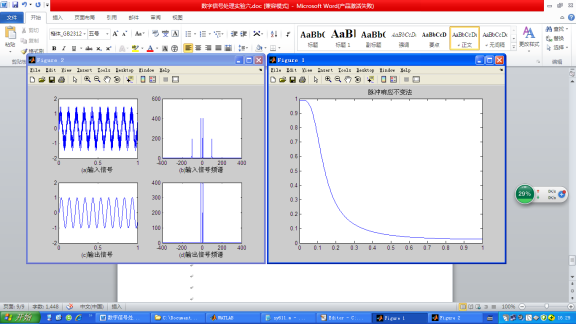4.假设一个信号x（t）=sin(2pif1t)+sin(2pif2t)+sin(2pif3*t),其中f1=200Hz，f2=1500Hz，f3=2900Hz。请设计一个IIR数字滤波器能把f2保留，请写出程序，并画出原信号与原信号通过滤波器的输出信号的图形。
（1）示例代码：

clear all
Wp=[0.2*pi 0.4*pi];
Ws=[0.1*pi 0.5*pi];
Ap=1;
As=25;
T=1;
Fs=1/T;
wp=(2/T)*tan(Wp/2);
ws=(2/T)*tan(Ws/2);
[N,wc]=cheb1ord(wp,ws,Ap,As,'s');
wc=wp/(10^(0.1*Ap)-1)^(1/2/N);
[num den]=butter(N,wc,'s');
[numd,dend]=impinvar(num,den,Fs);
w=linspace(0,pi,512);
h=freqz(numd,dend,w);
plot(w/pi,abs(h));
title('脉冲响应不变法');
%计算所设计滤波器的Ap和As
w1=[Wp,Ws];
h1=freqz(numd,dend,w1);
fprintf('Ap= %.4f\n',-20*log10(abs(h1(1))));
fprintf('As= %.4f\n',-20*log10(abs(h1(2))));
%信号传输
fs=800;
dt=1/fs;   %模拟信号采样间隔
f1=200;f2=1500;f3 = 2900;
t=0:dt:1;
x=sin(2*pi*f1*t)+sin(2*pi*f2*t)+sin(2*pi*f3*t);
y=filter(numd,dend,x); % 模拟输出
L=length(x);
x1=fftshift(fft(x));
ff=(-L/2:L/2-1)*fs/L;
y1=fftshift(fft(y));
figure(2)
subplot(2,2,1)
plot(t,x);
xlabel ('(a)输入信号');
subplot(2,2,2)
plot(ff,abs(x1));
xlabel ('(b)输入信号频谱');
subplot(2,2,3)
plot(t,y);
xlabel('(c)输出信号');
subplot(2,2,4)
plot(ff,abs(y1));
xlabel ('(d)输出信号频谱');


（2）运行结果：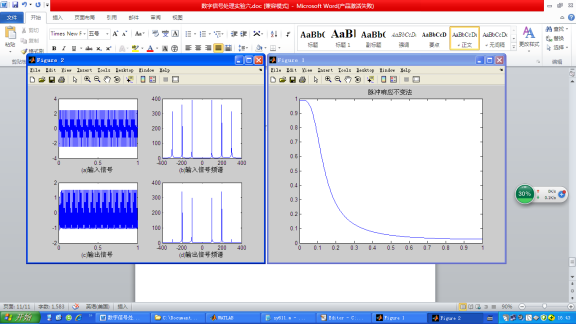# 更多相关文章点这里哦

数字信号处理----全套Matlab实验报告

通信工程(信息类，电子类，电气工程，自动化，计算机，软件工程，机电，等相关专业)全套学习指导答疑资料qq群:1007576722

展开全文算法
• 基于MATLAB GUI的IIR数字滤波器语音信号去噪处理平台的设计与实现 代码而已
• 本书以Altera公司的FPGA器件为开发平台，采用MATLAB及Verilog HDL语言为开发工具，详细阐述数字滤波器的FPGA实现原理、结构、方法以及仿真测试过程，并通过大量工程实例分析FPGA实现过程中的具体技术细节。...
• 这是一个用于设计 iir 滤波器matlab 程序，可以修改该程序以获得其他类型的滤波器
• 本次数字滤波器设计方法是基于MATLAB的数字滤波器的设计，是用学过的数字信号理论为依据，用MATLAB代码来实现的。通过IIR数字滤波器的设计过程，说明如何利用MATLAB来完成数字滤波器的设计。
• 1.学生自己运用MATLAB设计IIR数字低通滤波器。方法不限； 2.实现信号的滤波 实验步骤 参考巴特沃斯滤波器的实验原理《数字信号处理》第五章巴特沃斯数字滤波器的设计。 源代码： clc; Td=1;Fs=1/Td; Wp=0.2pi;Ws=0.3...

# 实验目的及要求

1.学生自己运用MATLAB设计IIR数字低通滤波器。方法不限；
2.实现信号的滤波

## 实验步骤

参考巴特沃斯滤波器的实验原理《数字信号处理》第五章巴特沃斯数字滤波器的设计。
源代码：

clc;
Td=1;Fs=1/Td;
Wp=0.2*pi;Ws=0.3*pi;
Rp=1;
As=15;
Omegap=2/Td*tan(Wp/2);
Omegas=2/Td*tan(Ws/2);
[N,Omegac]=buttord(Omegap,Omegas,Rp,As,'s');
[z0,p0,k0]=buttap(N);
[Bap,Aap]=zp2tf(z0,p0,k0);
[b,a]=lp2lp(Bap,Aap,Omegac);
[bz,az]=bilinear(b,a,Fs);
[H,W]=freqs(bz,az);
figure;
plot(W/pi,20*log10(abs(H)));
title('巴特沃斯滤波器')
xlabel('频率');
ylabel('增益(dB)');
f1=10;%第一个点频信号分量频率
f2=30;%第二个点频信号分量频率
f3=45;%第三个点频信号分量频率
fs=100;%采样率
T=2;%间隔长度
n=round(T*fs);%采样点个数
t=linspace(0,T,n);
y=cos(2*pi*f1*t)+cos(2*pi*f2*t)+cos(2*pi*f3*t)+randn(size(t));
figure;
subplot(2,1,1);
plot(t,y);
title('输入信号时域图像');
xlabel('t/s');
ylabel('V');
fft_y=fftshift(fft(y));
f=linspace(-fs/2,fs/2,n);
subplot(2,1,2);
plot(f,abs(fft_y));
title('输入信号频域图像');
xlabel('f/Hz');
ylabel('V');
axis([ 0 50 0 100]);
figure;
final=filter(bz, az, y);
subplot(2,1,1);
plot(t,final);
title( '滤波后信号波形时域波形' );
xlabel('t/s');
ylabel('V');
finall=fftshift(fft(final));
subplot(2,1,2);
plot(f,abs(finall));
title( '滤波后信号频域波形' );
axis([ 0 50 0 100]);
### 实验结果及分析

代码来自.

## 实验结果及分析接下来我们将参数做出一些改变
Wp=0.4pi, Ws=0.6pi, Rp=2, As=30可以明显观察到滤波后信号频域的波形相比之下发生了变化，也就是说滤波器的性能下降了！

## 总结

Wp，Ws：过渡带的起讫位置
Rp：通带内允许的最大衰减
As：阻带允许的最小衰减 参

考资料
1.

展开全文• 导出具有给定幅度和带宽的峰值滤波器的系数。 所有系数都按照 Zolzer 的描述计算DAFX 书籍（第 50 - 55 页）。 该算法假设一个常数 Q 项通过等式使用。 用法：[B,A] = 峰化(G, Fc, Q, Fs); G 是对数增益（以 dB 为...
• 数字信号处理第四次试验：IIR数字滤波器设计及软件实现前言一、实验目的二、实验原理与方法三、实验环境四、实验内容及步骤五、实验结果截图（含分析）六、思考题 前言 为了帮助同学们完成痛苦的实验课程设计，本...

# 前言

为了帮助同学们完成痛苦的实验课程设计，本作者将其作出的实验结果及代码贴至CSDN中，供同学们学习参考。如有不足或描述不完善之处，敬请各位指出，欢迎各位的斧正！

# 一、实验目的

（1）熟悉用双线性变换法设计IIR数字滤波器的原理与方法；
（2）学会调用MATLAB信号处理工具箱中滤波器设计函数（或滤波器设计分析工具fdatool）设计各种IIR数字滤波器，学会根据滤波需求确定滤波器指标参数。
（3）掌握IIR数字滤波器的MATLAB实现方法。
（4）通过观察滤波器输入输出信号的时域波形及其频谱，建立数字滤波的概念。

# 二、实验原理与方法

设计IIR数字滤波器一般采用间接法（脉冲响应不变法和双线性变换法），应用最广泛的是双线性变换法。基本设计过程是：①先将给定的数字滤波器的指标转换成过渡模拟滤波器的指标；②设计过渡模拟滤波器；③将过渡模拟滤波器系统函数转换成数字滤波器的系统函数。MATLAB信号处理工具箱中的各种IIR数字滤波器设计函数都是采用双线性变换法。第六章介绍的滤波器设计函数butter、cheby1、cheby2和ellip可以分别被调用来直接设计巴特沃斯、切比雪夫1、切比雪夫2和椭圆模拟和数字滤波器。本实验要求读者调用如上函数直接设计IIR数字滤波器。
本实验的数字滤波器的MATLAB实现是指调用MATLAB信号处理工具箱函数filter对给定的输入信号x(n)进行滤波，得到滤波后的输出信号y(n）。

# 三、实验环境

Matlab 7.0及Matlab 2018b

# 四、实验内容及步骤

（1）调用信号产生函数mstg产生由三路抑制载波调幅信号相加构成的复合信号st，该函数还会自动绘图显示st的时域波形和幅频特性曲线，如图所示。由图可见，三路信号时域混叠无法在时域分离。但频域是分离的，所以可以通过滤波的方法在频域分离，这就是本实验的目的。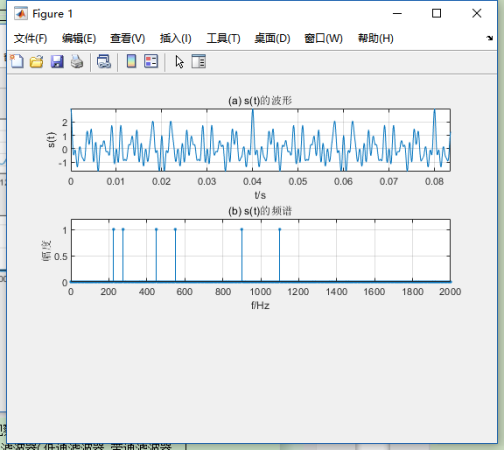（2）要求将st中三路调幅信号分离，通过观察st的幅频特性曲线，分别确定可以分离st中三路抑制载波单频调幅信号的三个滤波器（低通滤波器、带通滤波器、高通滤波器）的通带截止频率和阻带截止频率。要求滤波器的通带最大衰减为0.1dB,阻带最小衰减为60dB。
提示：抑制载波单频调幅信号的数学表示式为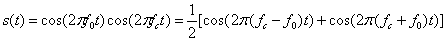其中， c o s ( 2 π f c t ) cos(2\pi f_ct) 称为载波， f c f_c 为载波频率， c o s ( 2 π f 0 t ) cos(2\pi f_0t) 称为单频调制信号， f 0 f_0 为调制正弦波信号频率，且满足 f c > f 0 f_c>f_0 。由上式可见，所谓抑制载波单频调幅信号，就是2个正弦信号相乘，它有2个频率成分：和频 f c + f 0 f_c+f_0 和差频 f c − f 0 f_c-f_0 ，这2个频率成分关于载波频率 f c f_c 对称。所以，1路抑制载波单频调幅信号的频谱图是关于载波频率 f c f_c 对称的2根谱线，其中没有载频成分，故取名为抑制载波单频调幅信号。容易看出，图中三路调幅信号的载波频率分别为250Hz、500Hz、1000Hz。如果调制信号m(t)具有带限连续频谱，无直流成分，则 s ( t ) = m ( t ) c o s ( 2 π f c t ) s(t)=m(t)cos(2\pi f_ct) 就是一般的抑制载波调幅信号。其频谱图是关于载波频率 f c f_c 对称的2个边带（上下边带），在专业课通信原理中称为双边带抑制载波(DSB-SC)调幅信号,简称双边带(DSB)信号。如果调制信号m(t)有直流成分，则 s ( t ) = m ( t ) c o s ( 2 π f c t ) s(t)=m(t)cos(2\pi f_ct) 就是一般的双边带调幅信号。其频谱图是关于载波频率 f c f_c 对称的2个边带（上下边带），并包含载频成分。
（3）编程序调用MATLAB滤波器设计函数ellipord和ellip分别设计这三个椭圆滤波器，并绘图显示其幅频响应特性曲线。
（4）调用滤波器实现函数filter，用三个滤波器分别对信号产生函数mstg产生的信号st进行滤波，分离出st中的三路不同载波频率的调幅信号 y 1 ( n ) y_1(n) y 2 ( n ) y_2(n) y 3 ( n ) y_3(n) ，并绘图显示 y 1 ( n ) y_1(n) y 2 ( n ) y_2(n) y 3 ( n ) y_3(n) 的时域波形，观察分离效果。

# 五、实验结果截图（含分析）

滤波器参数及实验程序清单
1、滤波器参数选取
观察图可知，三路调幅信号的载波频率分别为250Hz、500Hz、1000Hz。带宽（也可以由信号产生函数mstg清单看出）分别为50Hz、100Hz、200Hz。所以，分离混合信号st中三路抑制载波单频调幅信号的三个滤波器（低通滤波器、带通滤波器、高通滤波器）的指标参数选取如下：
对载波频率为250Hz的条幅信号，可以用低通滤波器分离，其指标为
带截止频率 f p f_p =280Hz，通带最大衰减 a p a_p =0.1dB；
阻带截止频率 f s f_s =450Hz，阻带最小衰减 a s a_s =60dB，
对载波频率为500Hz的条幅信号，可以用带通滤波器分离，其指标为
带截止频率 f p i f_{pi} =440Hz， f p u f_{pu} =560Hz，通带最大衰减 a p a_p =0.1dB；
阻带截止频率 f s i f_{si} =275Hz， f s u f_{su} =900Hz，阻带最小衰减 a s a_s =60dB，
对载波频率为1000Hz的条幅信号，可以用高通滤波器分离，其指标为
带截止频率 f p f_p =890Hz，通带最大衰减 a p a_p =0.1dB；
阻带截止频率 f s f_s =550Hz，阻带最小衰减 a s a_s =60dB，
说明：（1）为了使滤波器阶数尽可能低，每个滤波器的边界频率选择原则是尽量使滤波器过渡带宽尽可能宽。
（2）与信号产生函数mstg相同，采样频率Fs=10kHz。
（3）为了滤波器阶数最低，选用椭圆滤波器。
按照图4.2所示的程序框图编写的实验程序为exp4.m。

实验程序清单

%IIR数字滤波器设计及软件实现
clear;close all
Fs=10000;T=1/Fs;%采样频率
%调用信号产生函数mstg产生由三路抑制载波调幅信号相加构成的复合信号st
st=mstg;
%低通滤波器设计与实现=========================================
fp=280;fs=450;
wp=2*fp/Fs;ws=2*fs/Fs;rp=0.1;rs=60;%OF指标(低通滤波器的通、阻带边界频)
[N,wp]=ellipord(wp,ws,rp,rs);%调用ellipord计算椭圆DF阶数N和通带截止频率wp
[B,A]=ellip(N,rp,rs,wp);%调用ellip计算椭圆带通DF系统函数系数向量B和A
y1t=filter(B,A,st);%滤波器软件实现
%低通滤波器设计与实现绘图部分
figure(2);subplot(3,1,1);
myplot(B,A);%调用绘图函数myplot绘制损耗函数曲线
yt='y_1(t)';
subplot(3,1,2);tplot(y1t,T,yt);%调用绘图函数tplot绘制滤波器输出波形
%带通滤波器设计与实现=================================================
fpl=440;fpu=560;fsl=275;fsu=900;
wp=[2*fpl/Fs,2*fpu/Fs];ws=[2*fsl/Fs,2*fsu/Fs];rp=0.1;rs=60;
[N,wp]=ellipord(wp,ws,rp,rs);%调用e1lipord计算椭圆DF阶数N和通带截止频率wp
[B,A]=ellip(N,rp,rs,wp);%调用ellip计算椭圆带通DF系统函数系数向量B和A
y2t=filter(B,A,st);%滤波器软件实现
%带通滤波器设计与实现绘图部分
figure(2);subplot(3,1,1);
myplot(B,A);%调用绘图函数myplot绘制损耗函数曲线
yt='y_2(t)';
subplot(3,1,2);tplot(y2t,T,yt);axis([0,0.08,-1.2,1.2])%调用绘图函数tplot绘制滤波器输出波形
%高通滤波器设计与实现================================================
fp=890;fs=600;
wp=2*fp/Fs;ws=2*fs/Fs;rp=0.1;rs=60;%OF指标(低通滤波器的通、阻带边界频)
[N,wp]=ellipord(wp,ws,rp,rs);%调用ellipord计算椭圆DF阶数N和通带截止频率wp
[B,A]=ellip(N,rp,rs,wp,'high');%调用ellip计算椭圆带通DF系统函数系数向量B和A
y3t=filter(B,A,st);%滤波器软件实现
%高低通滤波器设计与实现绘图部分
figure(2);subplot(3,1,1);
myplot(B,A);%调用绘图函数myplot绘制损耗函数曲线
yt='y_3(t)';
subplot(3,1,2);tplot(y3t,T,yt);axis([0,0.08,-1.2,1.2])

function tplot(xn,T,yn)
n=0:length(xn)-1;
t=n*T;
plot(t,xn);
xlabel('t/s');
ylabel('yn');
axis([0,t(end),min(xn),1.2*max(xn)]);

function myplot(B,A)
[H,W]=freqz(B,A,1000);
m=abs(H);
plot(W/pi,20*log10(m/max(m)));
grid on;
xlabel('\omega/\pi');
ylabel('幅度(dB)')
axis([0,1,-80,5]);
title('损耗函数曲线');

function st=mstg
%产生信号序列向量st,并显示st的时域波形和频谱
%st=mstg返回三路调幅信号相加形成的混合信号，长度N=1600
N=1600%N为信号st的长度。
Fs=10000;T=1/Fs;Tp=N*T;%采样频率Fs=10kHz，Tp为采样时间
t=0:T:(N-1)*T;k=0:N-1;f=k/Tp;
fc1=Fs/10;%第1路调幅信号的载波频率fc1=1000Hz,
fm1=fc1/10;%第1路调幅信号的调制信号频率fm1=100Hz
fc2=Fs/20;%第2路调幅信号的载波频率fc2=500Hz
fm2=fc2/10;%第2路调幅信号的调制信号频率fm2=50Hz
fc3=Fs/40;%第3路调幅信号的载波频率fc3=250Hz,
fm3=fc3/10;%第3路调幅信号的调制信号频率fm3=25Hz
xt1=cos(2*pi*fm1*t).*cos(2*pi*fc1*t);%产生第1路调幅信号
xt2=cos(2*pi*fm2*t).*cos(2*pi*fc2*t);%产生第2路调幅信号
xt3=cos(2*pi*fm3*t).*cos(2*pi*fc3*t);%产生第3路调幅信号
st=xt1+xt2+xt3;%三路调幅信号相加
fxt=fft(st,N);%计算信号st的频谱
subplot(3,1,1)
plot(t,st);grid;xlabel('t/s');ylabel('s(t)');
axis([0,Tp/8,min(st),max(st)]);title('(a)s(t)的波形')
subplot(3,1,2)
stem(f,abs(fxt)/max(abs(fxt)),'.');grid;title('(b)s(t)的频谱')
axis([0,Fs/5,0,1.2]);
xlabel('f/Hz');ylabel('幅度')


实验程序运行结果及分析讨论
实验4程序运行结果如图所示。由图可见，三个分离滤波器指标参数选取正确，算耗函数曲线达到所给指标。分离出的三路信号y1(n)，y2(n)和y3(n)的波形是抑制载波的单频调幅波。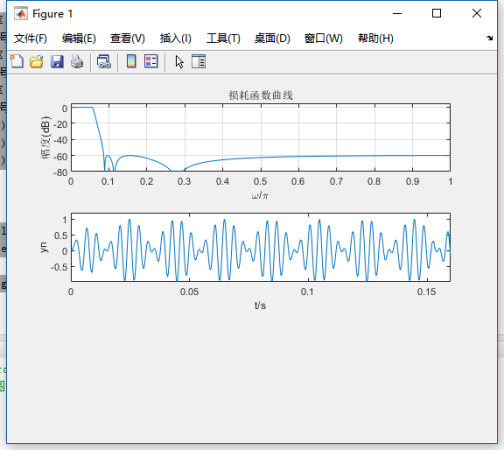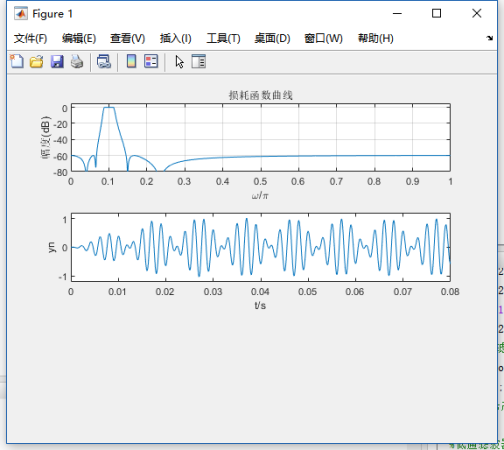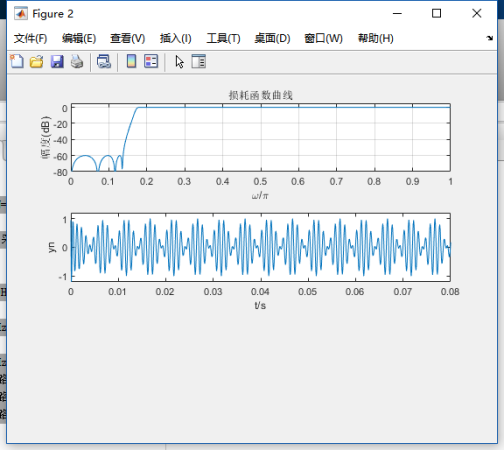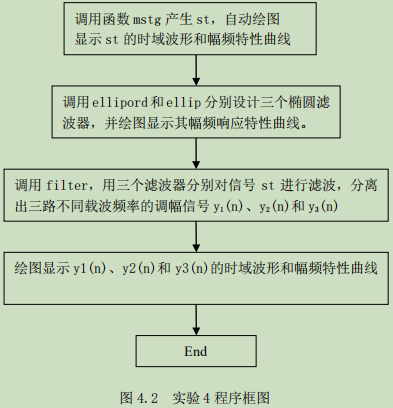# 六、思考题

（1）请阅读信号产生函数mstg，确定三路调幅信号的载波频率和调制信号频率。
（2）信号产生函数mstg中采样点数N=800，对st进行N点FFT可以得到6根理想谱线。如果取N=1000，可否得到6根理想谱线？为什么？N=2000呢？请改变函数mstg中采样点数N的值，观察频谱图验证您的判断是否正确。
（3）修改信号产生函数mstg，给每路调幅信号加入载波成分，产生调幅（AM）信号，重复本实验，观察AM信号与抑制载波调幅信号的时域波形及其频谱的差别。
提示：AM信号表示式： s ( t ) = [ 1 + c o s ( 2 π f 0 t ) ] c o s ( 2 π f c t ) s(t)=[1+cos(2\pi f_0t)]cos(2\pi f_ct)
答：（1）观察图可知，三路调幅信号的载波频率分别为250Hz、500Hz、1000Hz。带宽（也可以由信号产生函数mstg清单看出）分别为50Hz、100Hz、200Hz。所以，分离混合信号st中三路抑制载波单频调幅信号的三个滤波器（低通滤波器、带通滤波器、高通滤波器）的指标参数选取如下：
对载波频率为250Hz的条幅信号，可以用低通滤波器分离，其指标为
带截止频率 f p f_p =280Hz，通带最大衰减 a p a_p =0.1dB；
阻带截止频率 f s f_s =450Hz，阻带最小衰减 a s a_s =60dB，
对载波频率为500Hz的条幅信号，可以用带通滤波器分离，其指标为
带截止频率 f p i f_{pi} =440Hz， f p u f_{pu} =560Hz，通带最大衰减 a p a_p =0.1dB；
阻带截止频率 f s i f_{si} =275Hz， f s u f_{su} =900Hz，阻带最小衰减 a s a_s =60dB，
对载波频率为1000Hz的条幅信号，可以用高通滤波器分离，其指标为
带截止频率 f p f_p =890Hz，通带最大衰减 a p a_p =0.1dB；
阻带截止频率 f s f_s =550Hz，阻带最小衰减 a s a_s =60dB，
（3）因为信号st是周期序列，谱分析时要求观察时间为整数倍周期。所以，
本题的一般解答方法是，先确定信号st的周期，在判断所给采样点数N对应的观察时间Tp=NT是否为st的整数个周期。但信号产生函数mstg产生的信号st共有6个频率成分，求其周期比较麻烦，故采用下面的方法解答。
分析发现，st的每个频率成分都是25Hz的整数倍。采样频率Fs=10kHz=25×400Hz，即在25Hz的正弦波的1个周期中采样400点。所以，当N为400的整数倍时一定为st的整数个周期。因此，采样点数N=800和N=2000时，对st进行N点FFT可以得到6根理想谱线。如果取N=1000，不是400的整数倍，不能得到6根理想谱线。

展开全文• 2.内容：基于Matlab的fir和iir数字滤波器的设计与仿真包括matlab程序，word论文 ，PPT，程序操作视频 3.用处：用于fir和iir数字滤波器编程学习 4.指向人群：本硕博等学习教研使用,企事业简单项目方案验证参考
• 数字信号处理实验，包含代码跟实验截图，注释清晰明了，实验结果正确
• 学院 （系）： 电子信息与电气工程学部 专业： 电子信息工程 班级： 姓 名： 学号： 组： 实验时间： 实验室： 大黑楼C221 实验台： 指导教师签字： 成绩： 实验名称 实验三 IIR 数字滤波器设计 一、实验目的和要求 ...
• IIR：在MATLAB中，可以用下列函数辅助设计IIR数字滤波器：1)利用buttord和cheb1ord可以确定低通原型巴特沃斯和切比雪夫滤波器的阶数和截止频率；2)[num,den]=butter（N,Wn）和[num,den]=cheby1（N,Wn）,[num,den]=...
• （2）掌握IIR数字滤波器MATLAB实现方法设计各种滤波器。 （3）观察分析滤波器输入输出数据波形，理解数字滤波的概念。 二、实验原理 1、IIR数字滤波器设计的一般方法 IIR数字滤波器设计最常采用的方式是先按技术...
• 基于Matlab的FFT频谱分析及IIR数字滤波器设计.pdf
• Matlab数字滤波器的设计（IIR与FIR)（内含Matlab代码） FIR滤波器的优缺点 优点：相位线性度好，处理速度快，没有反馈回路稳定性强于IIR。 缺点：FIR幅频特性精度较之于IIRIIR滤波器 优点：相同阶数下IIR滤波效果...自动驾驶 机器学习
• 1.简介在做音频eq的时候，iir滤波器是必不可少的，所以这节主要学习一下iir滤波器，当然，不会偏于理论，而是着重怎么用。iir滤波器主要是用来处理信号的频率的，这是第一个最基本的认识。摆一下基本的公式：系统...
• 基于FPGA的FIR数字滤波器+matlab代码，verilog代码，word论文，基于quartusii平台开发。 第一章 数字滤波器原理及选择 2 1．1 数字滤波器简介 2 1．2 FIR与IIR数字滤波器 2 1．3 FIR与IIR数字滤波器的比较与选择 3...
• 首先IIR滤波器大家都知道，就是无限长脉冲序列滤波器。无限长是因为存在反馈，众所周知，存在反馈（负反馈，正反馈信号就不稳定了）系统更容易稳定，这个容易体现在滤波器的级数比较少，也就是说使用的元器件比较少...
• 数字滤波器是数字信号处理系统中重要的组成部分[1-3],一般分为有限冲激响应(Finite impulseresponse-FIR)滤波器和无限冲激响应(Infiniteimpulse response-IIR)滤波器两种.FIR滤波器在实际应用中有很多吸引人的特性,...
• 目录1. 概要2. IIR 和 FIR 滤波器的定义3. IIR滤波器和FIR滤波器的优缺点对比4. 双二次（Biquad）IIR 滤波器5. IIR滤波器设计指标（specifications）5.1 滤波器的频率响应特性5.2 模拟... 基于Matlab的SOS IIR滤波器...

# iir数字滤波器matlab代码matlab 订阅